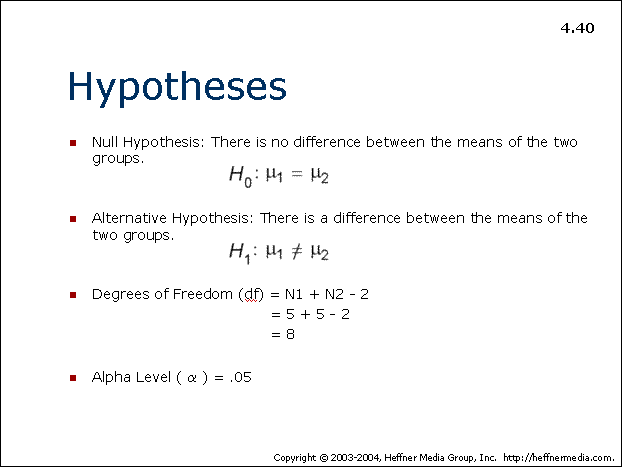# 40## Hypotheses

• Null Hypothesis: There is no difference between the means of the two groups
• H0 : μ1 = μ2

• Alternative Hypothesis: There is a difference between the means of the two groups.
• H1 : μ1 ≠ μ2

• Degrees of Freedom (df) = N1 + N2 – 2
•                                              = 5 + 5 – 2

= 8

• Alpha Level (α) = .05